# Flying

The airplane from Prague to Bratislava was flying at a speed of 60 km/h less and back by 70 km/h greater than the original speed. What was the original speed if the plane returned to Prague according to the timetable?

v =  840 km/h

### Step-by-step explanation: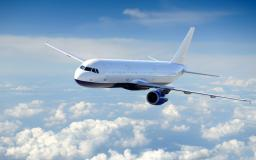Did you find an error or inaccuracy? Feel free to write us. Thank you!Tips to related online calculators
Looking for calculator of harmonic mean?
Looking for a statistical calculator?
Looking for help with calculating roots of a quadratic equation?
Do you have a linear equation or system of equations and looking for its solution? Or do you have a quadratic equation?
Do you want to convert velocity (speed) units?
Do you want to convert time units like minutes to seconds?

## Related math problems and questions:

• Express train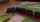International express train drove from Kosice to Teplice. In the first 279 km, the track was repaired, and therefore it was moving at a speed of 10km/h less than it was scheduled to drive. The rest of the 465 km trip has increased the speed by 8 km/h than
• Two citiesThe car goes from city A to city B at an average speed of 70 km/h, back at an average speed of 50 km/h. If it goes to B and back at an average speed of 60 km/h, the whole ride will take 8 minutes less. What is the distance between cities A and B?
• Forth and back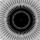Car drive from point A to point B at speed 78 km/h and back at 82 km/h. If went there and back at speed 81 km/h journey would take five minutes less. What is the distance between points A and B?
• Truck PRG-BA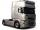Truck driving on the highway from Prague to Bratislava at an average speed of 72 km/h. At the moment when the truck passes 54 kilometers from Prague passenger car departs from Prague, which travels to Bratislava at a speed of 90 km/h. When and where (at w
• Average speed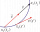The car drove on one section of the highway for half an hour at a speed of 80 km/h. Then he reduced his speed to 60km/h. It went three-quarters of an hour at a speed of 60 km/h. What was the average speed of the car?
• Average speedThe car drove from city A to city B at a 40 km/h and back to city A at a speed of 80 km/h. What was the average speed of the car?
• Car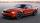The car goes from point A to point B at speed 86 km/h and back 53 km/h. If it goes there and back at speed 67 km/h trip would take 10 minutes shorter. What is the distance between points A and B?
• Speed of car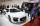The car went to a city that was 240 km away. If his speed increased by 8 km/h, it would reach the finish one hour earlier. Determine its original speed.
• Pedestrian up-down hill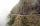Pedestrian goes for a walk first at the plane at 4 km/h, then uphill 3 km/h. Then it is in the middle of the route, turns back, and goes downhill at speed 6 km/h. The total walk was 6 hours. How many kilometers went pedestrian?
• Warehouse carsFrom the warehouse started truck at speed 40km/h. After 1hour 30mins started from the same place same direction a car at speed 70 km/h. For how long and at what distance from the warehouse overtake a truck?
• Average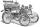What is the average speed of the car, where half of the distance covered passed at speed 66 km/h and the other half at 86 km/h.
• Two places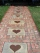The distance to the places is 60 km. From place A a pedestrian came out at a speed of 4 km/h and at the same time a car drove against him from place B. What was the speed of the car if the pedestrian met him in 90 minutes?
• Motorcyclist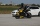From Trutnov, the motorcyclist started at an average speed of 60km/hour. At 12.30hrs the passenger car was started at a speed of 80km/hour. How many hours and at what distance from Trutnov will car catch a motorcycle?
• Between two bus stops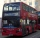Wanda lives between two bus stops at three-eighths of their distance. He started the house today and found that he would have arrived at the bus stop if he was running to one or the other stop. The average bus speed is 60 km/h. What is the average speed o
• Average speedThe truck drove 1/2 of the way on the highway at 80km/h. The other half of the way 20km/h. Calculate the average speed
• Double-track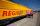On double-track express met with a freight train. Express travel at speed 72 km/h, a freight train at speed 36 km/h. After what time period will be from 9 km apart?
• Train expres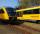At 8.00 strarted from Bratislava to Poprad express Tatry. Cities are distant 340 kilometers. At the same time started from Poprad express Danube to Bratislava. Express Tatry's average speed is 80 km/h, Danube 90 km/h. At what distance from Poprad trains w